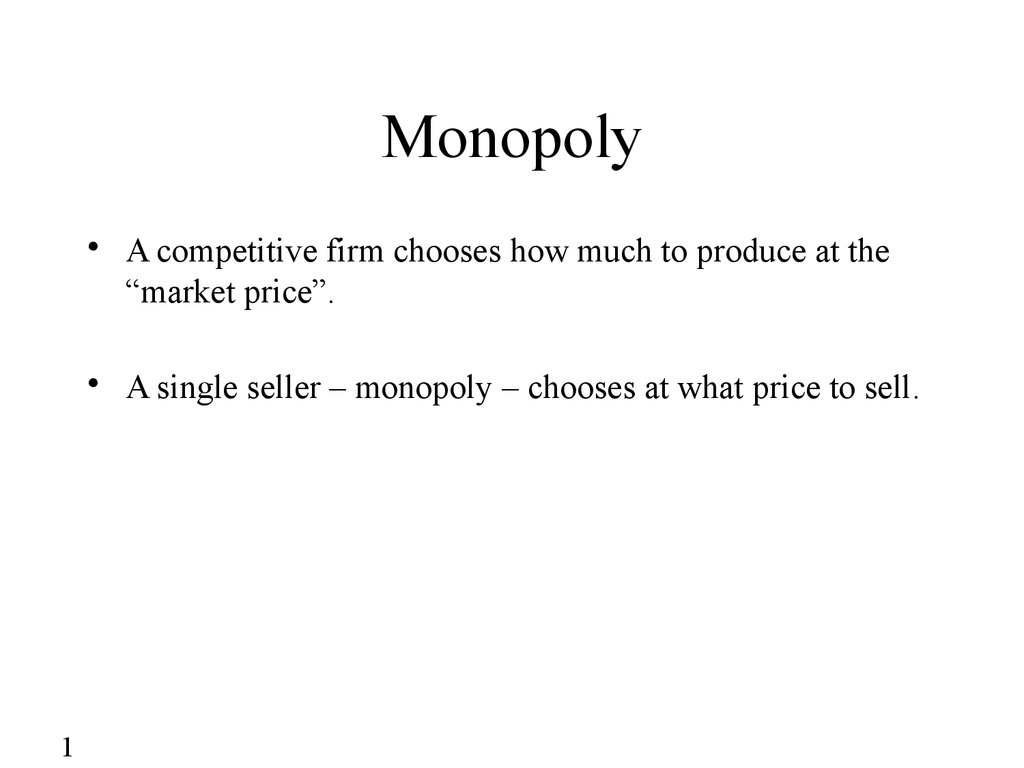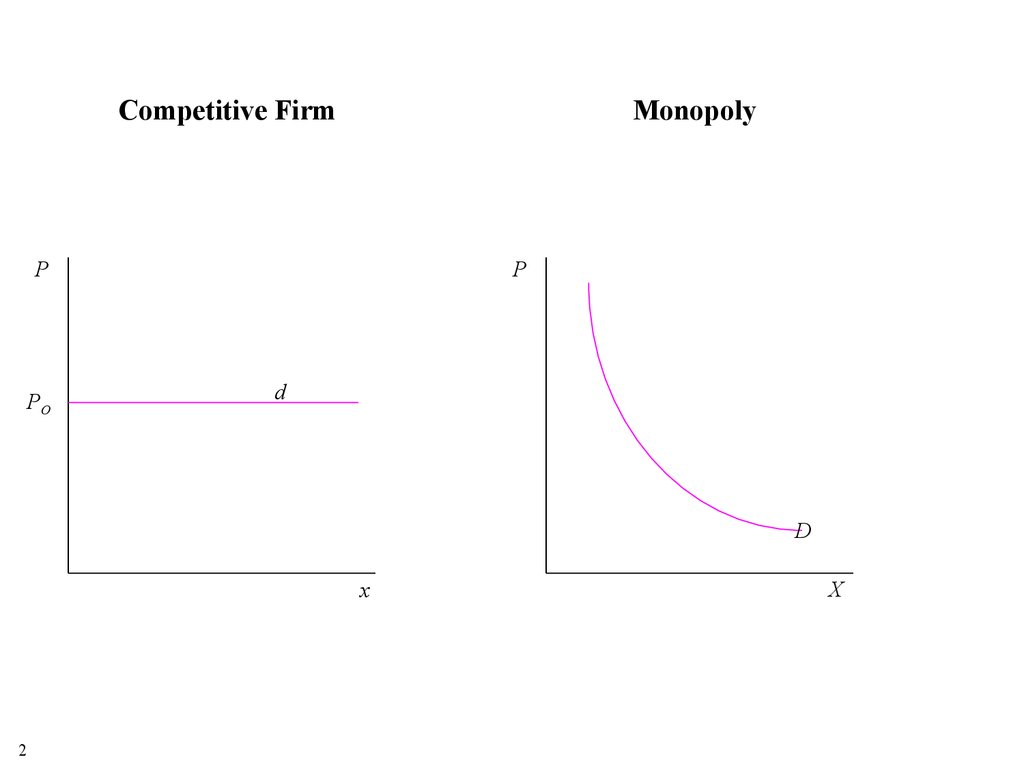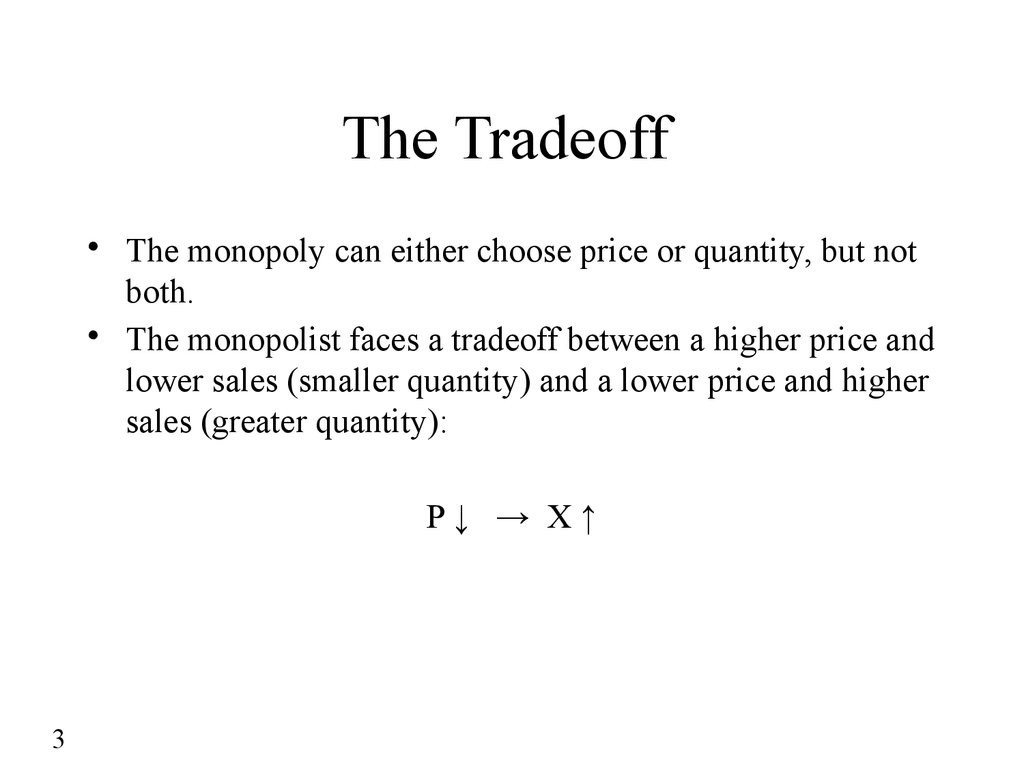Monopoly. Competitive firm monopoly

1. Monopoly

• A competitive firm chooses how much to produce at the
“market price”.
• A single seller – monopoly – chooses at what price to sell.
1

2.

Competitive Firm
Monopoly
P
PO
P
d
D
x
2
X

• The monopoly can either choose price or quantity, but not
both.
• The monopolist faces a tradeoff between a higher price and
lower sales (smaller quantity) and a lower price and higher
sales (greater quantity):
P↓ → X↑
3

4. Revenue

• Profit = Revenue – Total Costs
• Revenue = TR = Unit price • Quantity = P • X
• Increasing X is profitable if it increases Revenue
more than it increases Costs.
4

5. Marginal Revenue

• Marginal Revenue = MR= the change in total revenue
from increasing output by a unit.
• Marginal Cost = MC = the change in total costs from
increasing output by a unit.
• Increasing output (decreasing price) is profitable if MR is
greater than MC.
• Increasing output (decreasing price) is never profitable if
MR < 0.
5

6.

Example: X = 20 - 2P → P = 10 - 0.5X
TR
A
50
TR 10 X 0.5 X 2
P
20
X
P
10
TR PX
0
2
4
6
8
10
12
14
16
18
20
0
18
32
42
48
50
48
42
32
18
0
TR
X
18 / 2 9
14 / 2 7
10 / 2 5
3
1
–1
–3
–5
–7
–9
A
5
D : P 10 0.5 X
TR PX (10 0.5 X ) X 10 X 0.5 X 2
TR=50
10
6
10
9
8
7
6
5
4
3
2
1
0
X
MR
MR 10 X
20
X
dTR
MR 10 X
dX
MR 0
TR
MR 0
TR
MR 0
TR max